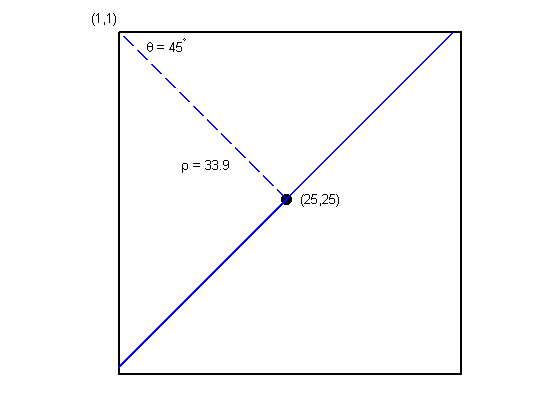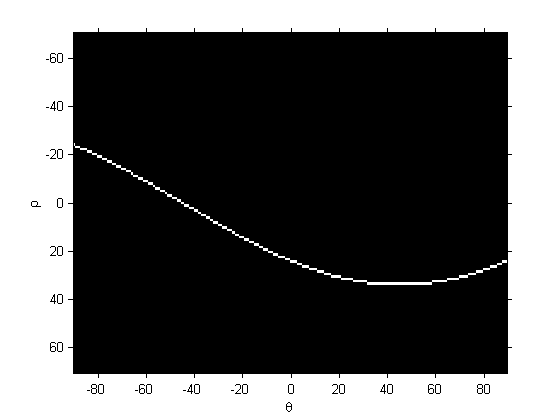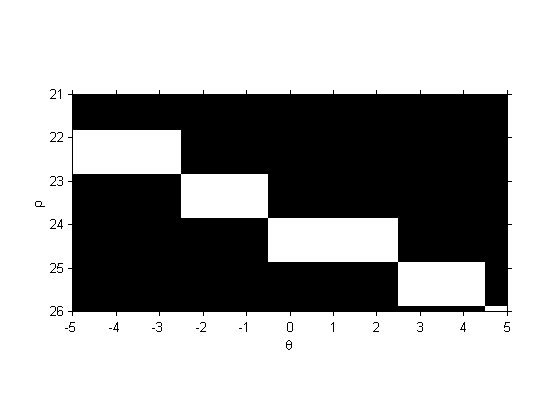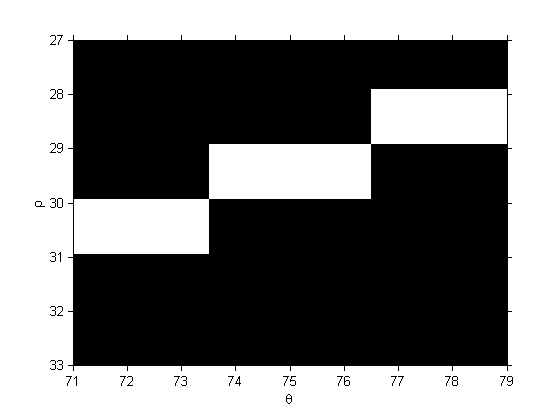# Hough transform coordinate system

Reader JP asked me about my recent blog post on the Hough transform. Specifically, she wanted to know exactly how the rho-theta coordinate system was defined. When I answered her question, I realized that our documentation isn't very clear on this point, so I thought I'd post the answer here.

Suppose you have a pixel at (x=25, y=25). (Note that the Image Processing Toolbox convention is that the x-axis is the horizontal axis, the y-axis is the vertical axis, and the origin is at the upper left.) There is a family of lines that all go through (25,25). The solid blue line in the diagram below is one such line. Draw a perpendicular projection from the upper left pixel to the line; that's the dashed blue line below. The length of the projection is rho. The clockwise angle between the projection and the horizontal axis is theta.

x = [1 50 50 1 1];
y = [1 1 50 50 1];
plot(x,y,'k','LineWidth',2,'Clipping','off')
axis equal
axis ij
axis off

hold on
plot(25,25,'k','Marker','o','MarkerSize',8,'MarkerFaceColor','k')

plot([1 25], [1 25], 'b', 'LineWidth', 2, 'LineStyle', '--')

plot([1 49], [49 1], 'b', 'LineWidth', 2)

text(10,20,'\rho = 33.9')
text(5,3,'\theta = 45^\circ')
text(27,25,'(25,25)')
text(-3,-1,'(1,1)')

hold offLet's try this with an image.

a = zeros(50,50);
a(25,25) = 1;
[H, theta, rho] = hough(a);

imshow(H, [], 'XData', theta, 'YData', rho, 'InitialMag', 'fit');
axis on
xlabel('\theta')
ylabel('\rho')Zooming in around the theta = 0 point gives us this:

axis([-5 5 21 26])There is a vertical line (theta = 0 degrees) that goes through the (25,25) pixel, and this line is 24 units away (perpendicular distance) from the upper left pixel.

Let's look at another spot.

axis([71 79 27 33])There is another line through (25,25) whose perpendicular projection from (1,1) is 75 degrees from the horizontal axis. The length of perpendicular projection to this line from (1,1) is about 29 units.